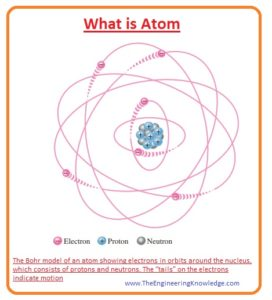Hello, fellows, I hope all of you are having fun in your life. In today’s tutorial, we will discuss What is Atom. Every type of matter created with the different types of atoms and atoms also consists of three main components first one is neutron second one is proton and the third one is electrons. Electrons in an atom revolve around the nucleus and proton and neutrons are exits in the center of the nucleus. In the periodic table, every element has a specific atom structure in an element all atoms have the same value for the protons numbers.

At the time of the invention of an atom, it was considered that atom is a single particle and no further particles exit in it. But with the passage of time, it was found that atom is not a single particle but it also comprises some subparts in its composition. In its center, there is a nucleus around which electrons are moving as in solar system planets are moving in a circular path. In today’s post, we will have a look at the atom’s structure, particles, and some related theories to an atom. So let’s get started with the What is Atom.

#### What is Atom

Bohr Model

• The atom is the least particle of any element that has the ability to take part in chemical reaction like elements.
• The atom of any element has the same properties and features shown by the element.
• Almost there are one hundred and eight elements that have been discovered that consist of different numbers of an atom all atoms of these elements are different from an atom of other elements.
• Due to the different features and structures of an atom, every element shows different behavior from other elements.
• The famous Neil Bohr who was famous physicists explains the structure that it is similar to our planet system in which all planets are revolving around the sun similarly in atoms all electrons are rotating around the nucleus.
• The nucleus of an atom comprises the two particles first one is the proton that has a positive charge and the second one is neutron that has zero charges.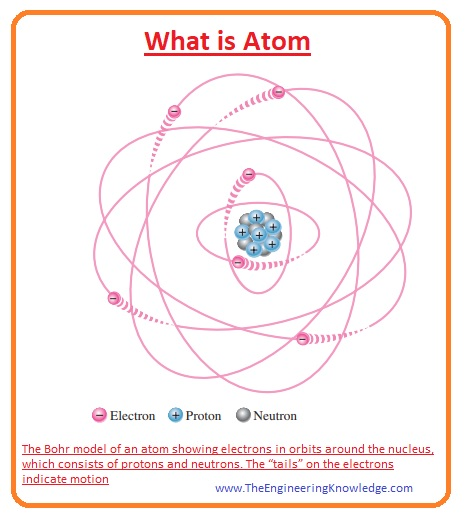• In every atom, there is a specific amount of protons and electrons that are not similar to other atoms of different elements.
• In given below figure you can see two different atoms first one is hydrogen and the second one is helium.
• You can see that hydrogen has one proton and one electron while helium has 2 protons (P) and 2 neutrons (N).• Atomic numbers of all the elements are shown on the periodic table.#### Atomic Number

• If we observe that all elements in the periodic table are placed in groups and periods of a table according to their atomic numbers.
• The number of protons in the nucleus of an atom defines the proton number of an atom.
• It is also equal to the number of electrons in such atoms that have the same numbers of electrons and protons such as an atom of hydrogen.
• Similarly in the atom of helium atomic number is 2 that is equal to the number of protons and electrons in the atom.

#### Electrons and Shells

Energy Levels

• The electrons of an atom revolve in specific orbit at a specific distance around atom according to the according to energy.
• The energy of electrons near to nucleus of an atom is less than the electrons that are away from the center of an atom.
• In atoms, there are different levels of energies so electrons revolve at different energy levels.
• Every orbit of an atom has a specific value of energy. In an atom, the orbits are grouped into energy levels called shells.
• Every atom has a specified value of shells and every shell have a certain quantity of electrons. These shells started from one and continue till the last number according to atoms and the number of electrons in an atom.
• In the given figure, you can see the atomic structure of silicon that has fourteen electrons and fourteen protons and neutrons in the nucleus.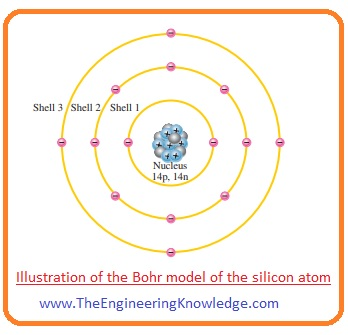How to find Maximum Number of electrons in a shell?

• The number of electrons in any shell can be denoted as Ne can find by using this given formula.

Ne =2n2

• In this formula, ‘n’ represents the number of shell of an atom. If we find number of atom in first shell than we will have.

Ne =2(1)2  = 2

• The number of electrons in the second shell will be.

Ne =2(2)2

=2×4=8

• The numbers of electrons in the third shell will be.

Ne =2(3)2

=2×9=18

• The number of electrons in the fourth shell will be.

Ne =2(4)2

=2×16=32

#### What is Valence Electrons

• The electrons in atom revolving in the last shell of an atom are called valence electrons.
• The energy of these electrons is larger as compared to other or less distant electrons from the nucleus.
• Due to the larger distance, the force of attraction of the nucleus on these electrons is also weaker.
• The last shell of an atom that has valence electrons called valence shell.
• The valence electrons take part in different chemical reactions and define the properties of the atom.
• Every electron in any shell requires a specific value of energy to leave their respective shell when valence electrons get the respective energy they left the shell and this ability explains the conductivity behavior of any material.

#### What is Ionization

• When the required energy of electrons in an atomic shell is provided either from any heating element or from the light they will leave their respective shells.
• The required energy depend on the distance from the nucleus if an electron is close to the nucleus it will require a large amount of energy due to a large force of attraction between nucleus and atom.
• If an electron is in valence shell it will need less amount of energy to leave the shell.
• The energy required for the electron to leave the shell is known as ionization energy.
• When electron leaves the atom at the place of electron positive ion is created or we can say a number of proton increases in the atom.
• The complete process of elimination of electron from the atom is called ionization.
• For instance, as we know the symbol of a hydrogen atom is H when outermost shell electron or valence shell electron will leave the atom positive ion will be created that is denoted as H+ the eliminated electron from the atom is known as the free electron.
• If instead of elimination of electron from atom it adds into atom this process creates negative ion and also releases energy from the atom.
• The removal or addition process of an electron does not happen in an only a single atom in different reactions more than one atom release or get electrons according to reaction requirements.

#### Quantum Model of Atom

• Normally the atomic model given by Bohr is widely used due to easy understanding but it does not provide some detailed explanation.
• Instead of the Bohr model quantum model is nowaday of an atom is think to detailed and correct atomic model.
• The quantum atomic model is a little bit complicated and difficult to understand as compared to the Bohr model.
•  For an understanding of a quantum model, there are two theories that explain the quantum model with the detailed.
• Wave-particle duality.
• Uncertainty principle.
• Now we discuss these two with the detailed.

Wave-Particle Duality

• This theory about electrons says that like light electron also shows two behavior of wave and like a particle.
• The speed with which the electron is moving in a its respective orbit represents its wavelength that interacts with the adjacent electron’s wave and adds up or cancels them at different points.

Uncertainty Principle

• As we are familiar with the behavior of waveform that continuously changes its behavior so according to the uncertainty principle, the position of an electron can not be specified.
• Uncertainty principle was given by Heisenberg, who says that it is very difficult to find the speed with which atom is moving and position at the same time with accuracy.
• As we discuss above quantum model is complex as compared to the Bohr in the sense that in quantum model every shell also further shell or subshells that are known as energy levels.
• These sub-shells are denoted as s,p, d, and f. In s sub-shell, only 2 electrons can reside.
• P has 6 electrons, d has 10 and f has 14 electrons.
• Every atom can be explained with the atom arrangements that have shell and subshell and electron in these subshells according to their position.
•  For instance, in a given table you can see the nitrogen configuration and electrons distribution in shells.
 Notation Explanation 1s2 There are two electrons in the first shell and s orbital or subshell. Here 1 denotes the first shell. And s is a subshell 2s2 2p3 In second shell there are five electrons that are two in s orbital and three in p orbital or subshell of the second shell
•  The structure of orbitals or subshells is not like the shells explained in the Bohr model.  In the quantum model, every shell in the Bohr model is a 3-dimensional space covering the atom that denotes the average value of the energy of the electronic cloud.
• The electronic clouds term is used about the area around the electrons that shows the electron’s existence.
• In a 3-D representation of a quantum model of atom S subshell or orbitals, the shape is similar to the sphere.
• For the first energy level, the structure of the sphere is solid while in higher energy levels every s subshell consists of spherical shapes which are nested shells.
• The structure of p subshell in the second shell has 2 ellipse shape lobes also have tangent point on the nucleus.
• The 3 p subshells in every shell are located at 900 to each other. One p subshell at the x-axis, second at y-axis and third at z-axis.
• For instance, the quantum model of sodium atom consists of eleven electrons is drawn in given figure below.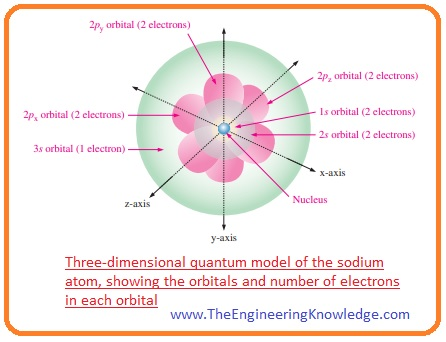#### Difference between Atoms and Moles

• Now we compare the atom and moles and find their differences.
 Atom Moles Atom is the basic element of matter and takes part in the construction of matter. While mole is the unit that indicates the quantity of any substance in a chemical reaction. Atom is the least particle of matter that shows the complete behavior of an element. Mole is the unit that tells about the quantity of particles in any substance with comparing to the particles exits in twelve grams of carbon 12. Molecules to atom conversion do not always happen. Converting to moles is a little bit easy as the quantity remains the same. Atoms consist of protons, electrons, and neutrons. Mole is represented with the Avogadro’s numbers.

#### Differences between Atoms and Molecules

• Now we discuss the difference between atom and molecules.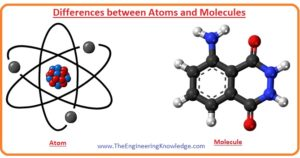Atom Molecules Atom is the smallest particle of an element. Molecules are a combination of two or more atoms. The example of different atoms is oxygen (O), hydrogen (H), phosphorus (P). Examples of molecules are O2, H2, H2O. It is not stable due to the elimination and addition of atoms. Molecules remain stable. Its Constituent particles are protons, electrons, and neutrons. Molecules are created with the atom of the same type or different. Almost all atoms take parts in chemical reactions except noble gases atoms. While molecules due to the absence of valence electrons do not take part in reactions without certain conditions.

That is the detailed post on atom I have mentioned each and every parameter related to an atom. If you have any questions about this tutorial ask in comments. See you in the next tutorial.

1. hgf says:

Great weblog here! Also your web site lots up very fast!
What host are you the usage of? Can I am getting your

2. xsex1tube.xyz says: